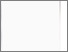# Investigation of a digital FEM height reference surface as vertical reference system

Schneid, S., 2007. Investigation of a digital FEM height reference surface as vertical reference system. PhD, Nottingham Trent University.Preview
Text
10183209.pdf - Published version

## Abstract

In recent years, the number of precise online DGNSS (Differential Global Navigation Satellite System) applications has significantly increased. Precise DGNSS correction services have been created that enable an online positioning with accuracy in the centimetre region, hi contrast to the co-ordinates found by DGNSS, the measured height needs to be transformed. This is because national height systems refer to a physically defined Height Reference Surface, HRS, that approximates the mean sea level, while the height derived from DGNSS positioning is the height above the WGS84 (World Geodetic System 1984), a mathematical model of the earth and is therefore called "ellipsoidal height". So for the application of precise DGNSS services and for the generation of transformation messages, such as RTCM 3.0, there is an urgent need for a HRS, in a unified datum with appropriate accuracy.

This thesis deals with the concept of the Digital FEM (Finite Element Method) Height Reference Surface, DFHRS. This concept enables the rigorous least squares adjustment of any HRS related observation. The HRS is modelled as continuous surface by a local Taylor-series expansion in a grid of FEM-meshes. With this, areas of any size may be computed. The theory of the DFHRS and further development of the mathematical model, especially the incorporation of observed gravity accelerations, are the main parts of this thesis.

As the applied Taylor-series expansion of the DFHRS concept only holds for a two- dimensional approximation. Spherical Cap Harmonics, SCH, had to be introduced as auxiliary parameter, to give a complete representation of the local gravity field. Spherical Cap Harmonics, SCH, may be interpreted as the general case of Spherical Harmonics, SH, that have been applied in geodetic applications for decades. The goal of the SCH coefficients, in contrast to the SH coefficients is that they may be applied over areas with limited extent. Due to numerical reasons, the combined least squares estimation of the DFHRS and the SCH coefficients in practical computations had to be done applying a sequential procedure.

In practical examples, different HRS representations with centimetre accuracy were computed by combining GNSS/Levelling points and precise gravimetric geoid models according to the DFHRS concept.

In a further example, gravity acceleration observations and a global geopotential model have been introduced into a combined least squares estimation of SCH coefficients. It could be shown that the introduction of the gravity accelerations leads to a significant improvement of the representation of the local gravity field. To perform a two step adjustment, height anomalies were derived from the determined SCH coefficients and introduced together with GNS S/Levelling points into a second adjustment according to the DFHRS concept. By comparison with a reference model, the resulting accuracy of the HRS representation was estimated to be 0.025m.

Item Type: Thesis
Creators: Schneid, S.
Date: 2007
ISBN: 9781369314755
Identifiers:
NumberType
PQ10183209Other
Divisions: Schools > School of Science and Technology
Record created by: Linda Sullivan
Date Added: 22 Sep 2020 07:17Edit View